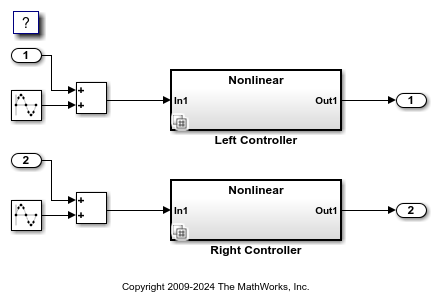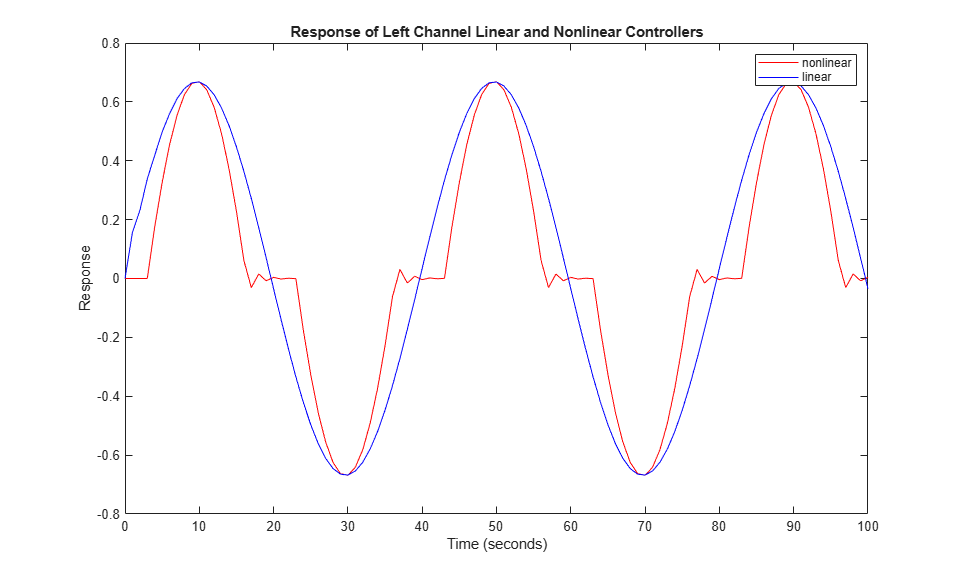# Use Variant Models to Generate Code That Uses C Preprocessor Conditionals

This example shows you how to use variant models to generate code that uses preprocessor conditionals to control which code is linked into the embedded executable.

### Overview of Variant Models

You can use a Model block to reference one Simulink® model (the child model) from another Simulink® model (the parent model). A Variant Subsystem block can have different variants. The variants can include a set of Model blocks, from which the Variant Subsystem block selects one. The figure below is a conceptual depiction of variant models. In this example the `Right Controller` model block can potentially select from two Model blocks. The models referenced by the Model blocks provide variations upon a nominal, prescribed functionality.A Variant Subsystem block has only one variant active at a time. You can use the Variant Subsystem block dialog to select the active variant. Alternatively, you can parameterize the selection of the active variant, and make it dependent on the values of variables and objects in the base MATLAB® workspace. When you generate code, you can generate code for all variants and defer the choice of active variant until it is time to compile that code.

### Specifying Variant Models

Opening the example model `rtwdemo_preprocessor` runs the PostLoadFcn defined in the "File: ModelProperties: Callbacks" dialog. This populates the base workspace with the control variables for the Variant Subsystem blocks.

```open_system('rtwdemo_preprocessor') ```The `Left Controller` variant subsystem contains two child Model blocks: `Linear` and `Nonlinear`. The `Left Controller/Linear` child model executes when the `Simulink.Variant` object `LINEAR` evaluates to `true`, and the `Left Controller/Nonlinear` child model executes when the `Simulink.Variant` object `NONLINEAR` evaluates to `true`.

`Simulink.Variant` objects are specified for the `Left Controller` subsystem by right-clicking the `Left Controller` subsystem and selecting `Subsystem Parameters`, which opens the `Left Controller` Variant Subsystem block dialog box.The `Left Controller` subsystem block dialog creates an association between the `Linear` and `Nonlinear` Model blocks with the two `Simulink.Variant` objects from the base workspace, `LINEAR` and `NONLINEAR`. These objects have a property named `Condition`, which is an expression that evaluates to a Boolean value and determines the active variant model (`Linear` or `Nonlinear`). The condition is also shown in the subsystem block dialog. In this example, the conditions of `LINEAR` and `NONLINEAR` are `'VSSMODE == 0'` and `'VSSMODE == 1'`, respectively.In this example, `Simulink.Variant` objects are created in the base workspace.

```LINEAR = Simulink.Variant; LINEAR.Condition = 'VSSMODE==0'; NONLINEAR = Simulink.Variant; NONLINEAR.Condition = 'VSSMODE==1'; ```

### Specifying a Variant Control Variable

Variant objects allow you to reuse arbitrarily complex conditions throughout a model. Multiple Variant Subsystem blocks can use the same `Simulink.Variant` objects, allowing you to toggle the activation of variant models as a set. You can toggle the set prior to simulation by changing the value of `VSSMODE` in the MATLAB environment or when compiling the generated code, as explained in the next section. In this example, `Left Controller` and `Right Controller` reference the same variant objects, so that you can toggle them simultaneously.

The nonlinear controller models implement hysteresis, while the linear controller models act as simple low-pass filters. Open the subsystem for the left channel. The models for the right channel are similar.

The generated code accesses the variant control variable `VSSMODE` as a user-defined macro. In this example, `rtwdemo_importedmacros.h` supplies `VSSMODE`. Within the MATLAB environment, you specify `VSSMODE` using a `Simulink.Parameter` object. Its value will be ignored when generating code including preprocessor conditionals. However, the value is used for simulation. The legacy header file specifies the value of the macro to be used when compiling the generated code, which ultimately activates one of the two specified variants in the embedded executable.

Variant control variables can be defined as `Simulink.Parameter` objects with one of these storage classes:

• `Define` or `ImportedDefine` with header file specified

• `CompilerFlag`

• `SystemConstant` (AUTOSAR)

• User-defined custom storage class that defines data as a macro in a specified header file

```VSSMODE = Simulink.Parameter; VSSMODE.Value = 1; VSSMODE.DataType = 'int32'; VSSMODE.CoderInfo.StorageClass = 'Custom'; VSSMODE.CoderInfo.CustomStorageClass = 'ImportedDefine'; VSSMODE.CoderInfo.CustomAttributes.HeaderFile = 'rtwdemo_importedmacros.h'; ```

### Simulating the Model with Different Variants

Because you set the value of `VSSMODE` to `1`, the model uses the nonlinear controllers during simulation.

```sim('rtwdemo_preprocessor') youtnl = yout; ```

If you change the value of `VSSMODE` to `0`, the model uses the linear controllers during simulation.

```VSSMODE.Value = int32(0); sim('rtwdemo_preprocessor') youtl = yout; ```

You can plot and compare the response of the linear and nonlinear controllers:

```figure('Tag','CloseMe'); plot(tout, youtnl.signals(1).values, 'r-', tout, youtl.signals(1).values, 'b-') title('Response of Left Channel Linear and Nonlinear Controllers'); ylabel('Response'); xlabel('Time (seconds)'); legend('nonlinear','linear') axis([0 100 -0.8 0.8]); ```### Using C Preprocessor Conditionals

This example model has been configured to generate C preprocessor conditionals. To generate code for the model, in C Code tab of the toolstrip select Build.

To activate code generation of preprocessor conditionals, check whether the following conditions are true:

• Select an Embedded Coder® target in Code Generation > System target file in the Configuration Parameters dialog box

• In the Variant Subsystem block parameter dialog box, set the Variant activation time parameter to `code compile`.

In this example, the generated code includes references to the `Simulink.Variant` objects `LINEAR` and `NONLINEAR`, as well as the definitions of macros corresponding to those variants. Those definitions depend on the value of `VSSMODE`, which is supplied in an external header file `rtwdemo_importedmacros.h`. The active variant is determined by using preprocessor conditionals (`#if`) on the macros (`#define`) `LINEAR` and `NONLINEAR`.

The macros `LINEAR` and `NONLINEAR` are defined in the generated `rtwdemo_preprocessor_types.h` header file:

``` |#ifndef LINEAR| |#define LINEAR (VSSMODE == 0)| |#endif|```
``` |#ifndef NONLINEAR| |#define NONLINEAR (VSSMODE == 1)| |#endif|```

In the generated code, the code related to the variants is guarded by C preprocessor conditionals. For example, in `rtwdemo_preprocessor.c`, the calls to the step and initialization functions of each variant are conditionally compiled:Close the model, figure, and workspace variables from the example.

```bdclose('rtwdemo_preprocessor') close(findobj(0,'Tag','CloseMe')); clear LINEAR NONLINEAR VSSMODE clear tout yout youtl youtnl ```